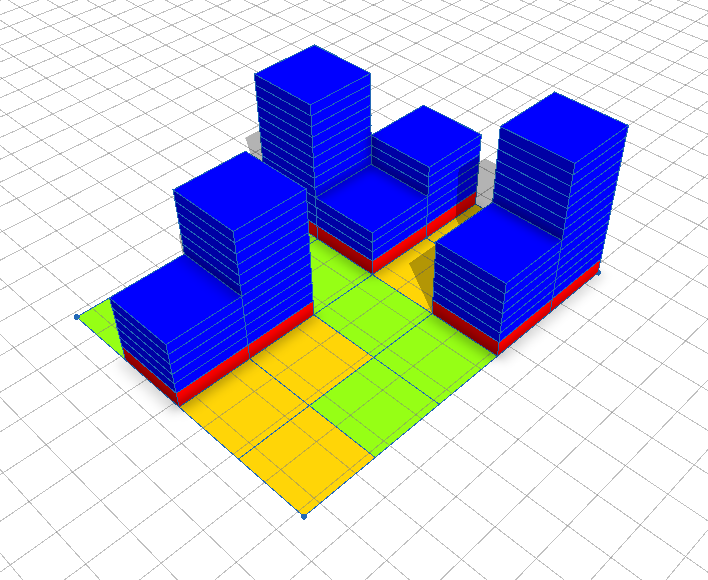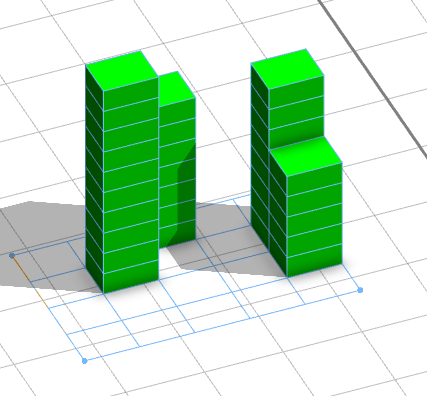# Random Heights

1020
2
12-10-2017 11:06 PMNew Contributor II

Hi,

I have split my parcel into different part and extruded building with 30% case, as shown in imageIs there a way where I can randomize the height with constant number of floors. For example, I have total number of floors 30, and I want to randomize the number of floors between 5(min floors) to 10(max floors) floors, In end the total number of floors should be 30. Similarly having control like first building lot should be with number of floors 10 and then decreasing with total number of floors 30.

Thanks

Tags (3)
2 RepliesbyEsri Regular Contributor

Thank you for this interesting question. If you want an exact number of floors, you need to influence the random floor number generation. Therefore you need to keep track of all floors generated. In a regular split operation this information is unavailable. The only way I achieved this, is by using a recursive tile generation.``````version "2017.1"

const floorHeight = 3

const minFloors = 5
const maxFloors = 10
const totalFloors = 30

attr floors = 0
attr floorsRemaining = totalFloors

const parcelsX = 4
const parcelsZ = 5
attr parcelsRemaining = parcelsX * parcelsZ

// const probabiltity = totalFloors / (parcelsX * parcelsZ * (minFloors+maxFloors)/2)
const probabiltity = 0.3

getFloorHeight =
case parcelsRemaining == 0: floorsRemaining
case floorsRemaining/parcelsRemaining > maxFloors: min(maxFloors, floorsRemaining-minFloors)
case floorsRemaining <= maxFloors: float(p(probabiltity)) * floorsRemaining
else: float(p(probabiltity)) * floor(rand(minFloors, min(maxFloors, floorsRemaining-minFloors)))

Lot -->
s(scope.sx/parcelsX,0,scope.sz/parcelsZ)
SplitXZ(0,0)

SplitXZ(i,j) -->
case i<parcelsX:
set(parcelsRemaining, parcelsRemaining-1)
set(floors, getFloorHeight)
set(floorsRemaining, floorsRemaining - floors)
Floors
t(scope.sx,0,0)
SplitXZ(i+1,j)
else:
case j<parcelsZ-1:
t(-scope.sx*parcelsX,0,scope.sz)
SplitXZ(0,j+1)
else: NIL

Floors -->
extrude(floors*floorHeight)
report("floors."+str(floors), 1)
split(y) {floorHeight: Color}*

Color -->
case floors > maxFloors: color(1,0,0)
case floors < minFloors: color(0,0,1)
else: color(0,1,0)
‍‍‍‍‍‍‍‍‍‍‍‍‍‍‍‍‍‍‍‍‍‍‍‍‍‍‍‍‍‍‍‍‍‍‍‍‍‍‍‍‍‍‍‍‍‍‍‍‍‍‍‍``````New Contributor II

Thanks Thomas, your code was really helpful to understand various ways to use functions.

I tried this code and works perfectly, but since my plots are getting divided base on the plot dimension say, 30*40, so I am not able to figure out how to incorporate this code.

Basically I split my parcels in grids using split function and generate give 30% probability to cover 30% ground cover for buildings and then randomize heights between max floors and min floors.

Since I am working with FAR concepts now I have total number of floors fixed. I hope we can incorporate your code in mine.

Here is my code

getHeight(row_ind, nRows, col_ind, nCols) = GfHeight + nFloors(row_ind, nRows, col_ind, nCols)*UfHeight

nFloors(row_ind, nRows, col_ind, nCols) =
case Variation_Mode == "None" || (nRows*nCols) < 2 : Floor_Max
case Variation_Mode == "Increasing" : Floor_Min + (rint((Floor_Max-Floor_Min)*((row_ind*nCols+col_ind)/((nRows*nCols)-1))))
case Variation_Mode == "Decreasing" : Floor_Min + rint((Floor_Max-Floor_Min)*(1-(row_ind*nCols+col_ind)/((nRows*nCols)-1)))
case Variation_Mode == "Alternating" : Floor_Min + rint((Floor_Max-Floor_Min)*((row_ind*nCols+col_ind)%2))
case Variation_Mode == "Plot_Increasing" : Floor_Min + (rint((Floor_Max-Floor_Min)*(col_ind/(nCols-1))))
# case Variation_Mode == "Plot_Decreasing" : Floor_Min + (rint((Floor_Max-Floor_Min)*((1-(col_ind))/(nCols-1)))) ///// Not Working
case Variation_Mode == "Plot_Alternative" : Floor_Min + (rint((Floor_Max-Floor_Min)*(col_ind%2)))
else : rint(rand(Floor_Min-0.49,Floor_Max+0.49))

@StartRule

Lot -->
set(Plot_Area, geometry.area)
report("Plot Area", Plot_Area)
Lot2

Lot2 -->
split(x) { Width: Col(split.index, split.total)}*

Col(col_ind, nCols) -->
split(z) { Length: Cell(split.index, split.total, col_ind, nCols)}*

Cell(row_ind, nRows, col_ind, nCols) -->
print("total cels " + (nRows*nCols))
print("row_ind " + (row_ind*nCols+col_ind))
DevelopLot(row_ind, nRows, col_ind, nCols)

DevelopLot(row_ind, nRows, col_ind, nCols) -->
case p(BuildPer/100) : ExtrudeBuild(row_ind, nRows, col_ind, nCols)
case p(OpenSpacePer/100) : Openspace
case p(GreenspacePer/100) : Greenspace
else : Greenspace

ExtrudeBuild(row_ind, nRows, col_ind, nCols) -->
extrude(world.y, getHeight(row_ind, nRows, col_ind, nCols))
split(y){GfHeight : Done. | ~1 : UpperFloors}

UpperFloors -->
split (y){~UfHeight : Done.}*